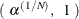# The NPAR1WAY Procedure

#### Exact Tests

PROC NPAR1WAY provides exact p-values for tests for location and scale differences based on the following scores: Wilcoxon, median, van der Waerden (normal), Savage, Siegel-Tukey, Ansari-Bradley, Klotz, Mood, and Conover. Additionally, PROC NPAR1WAY provides exact p-values for tests that use the raw data as scores. Exact tests are available for two-sample and multisample data. When the data are classified into two samples, tests are based on simple linear rank statistics. When the data are classified into more than two samples, tests are based on one-way ANOVA statistics.

Exact tests can be useful in situations where the asymptotic assumptions are not met and the asymptotic p-values are not close approximations for the true p-values. Standard asymptotic methods involve the assumption that the test statistic follows a particular distribution when the sample size is sufficiently large. When the sample size is not large, asymptotic results might not be valid, with the asymptotic p-values differing perhaps substantially from the exact p-values. Asymptotic results might also be unreliable when the distribution of the data is sparse, skewed, or heavily tied. For more information, see Agresti (2007) and Bishop, Fienberg, and Holland (1975). Exact computations are based on the statistical theory of exact conditional inference for contingency tables, reviewed by Agresti (1992).

In addition to computation of exact p-values, PROC NPAR1WAY provides the option of estimating exact p-values by Monte Carlo simulation. This can be useful for problems that are so large that exact computations require a great amount of time and memory, but for which asymptotic approximations might not be sufficient.

The following sections summarize the exact computational algorithms, define the exact p-values that PROC NPAR1WAY computes, discuss the computational resource requirements, and describe the Monte Carlo estimation option.

##### Computational Algorithms

PROC NPAR1WAY computes exact p-values by using the network algorithm developed by Mehta and Patel (1983). This algorithm provides a substantial advantage over direct enumeration, which can be very time-consuming and feasible only for small problems. See Agresti (1992) for a review of algorithms for computation of exact p-values, and see Mehta, Patel, and Tsiatis (1984) and Mehta, Patel, and Senchaudhuri (1991) for information about the performance of the network algorithm.

PROC NPAR1WAY constructs a contingency table from the input data, with rows formed by the levels of the classification variable and columns formed by the response variable values. The reference set for a given contingency table is the set of all contingency tables with the observed marginal row and column sums. Corresponding to this reference set, the network algorithm forms a directed acyclic network consisting of nodes in a number of stages. A path through the network corresponds to a distinct table in the reference set. The distances between nodes are defined so that the total distance of a path through the network is the corresponding value of the test statistic. At each node, the algorithm computes the shortest and longest path distances for all the paths that pass through that node. For the two-sample linear rank statistics, which can be expressed as linear combinations of cell frequencies multiplied by increasing row and column scores, PROC NPAR1WAY computes shortest and longest path distances by using the algorithm given by Agresti, Mehta, and Patel (1990). For the multisample one-way test statistics, PROC NPAR1WAY computes an upper bound for the longest path and a lower bound for the shortest path by following the approach of Valz and Thompson (1994).

The longest and shortest path distances (bounds) for a node are compared to the value of the test statistic to determine whether all paths through the node contribute to the p-value, none of the paths through the node contribute to the p-value, or neither of these situations occurs. If all paths through the node contribute, the p-value is incremented accordingly, and these paths are eliminated from further analysis. If no paths contribute, these paths are eliminated from the analysis. Otherwise, the algorithm continues, still processing this node and the associated paths. The algorithm finishes when all nodes have been accounted for.

In applying the network algorithm, PROC NPAR1WAY uses full numerical precision to represent all statistics, row and column scores, and other quantities involved in the computations. Although it is possible to use rounding to improve the speed and memory requirements of the algorithm, PROC NPAR1WAY does not do this because it can result in reduced accuracy of the p-values.

##### Definition of p-Values

For two-sample linear rank tests, PROC NPAR1WAY computes exact one-sided and two-sided p-values for each test that you specify in the EXACT statement. For one-sided tests, PROC NPAR1WAY displays the right-sided p-value when the observed value of the test statistic is greater than its expected value. The right-sided p-value is the sum of probabilities for those tables with a test statistic that is greater than or equal to the observed test statistic. Otherwise, when the observed test statistic is less than or equal to its expected value, PROC NPAR1WAY displays the left-sided p-value. The left-sided p-value is the sum of probabilities for those tables with a test statistic that is less than or equal to the observed value. The one-sided p-value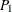can be expressed as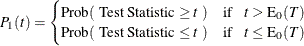where t is the observed value of the test statistic and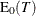is the expected value of the test statistic under the null hypothesis. PROC NPAR1WAY computes the two-sided p-value as the sum of the one-sided p-value and the corresponding area in the opposite tail of the distribution of the statistic, equidistant from the expected value. The two-sided p-value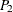can be expressed as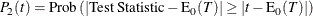Tests for multisample data are based on one-way ANOVA statistics. For a test of this form, large values of the test statistic indicate a departure from the null hypothesis; the test is inherently two-sided. The exact p-value is the sum of probabilities for those tables having a test statistic greater than or equal to the value of the observed test statistic.

If you specify the POINT option in the EXACT statement, PROC NPAR1WAY provides point probabilities for the exact tests. The point probability is the exact probability that the test statistic equals the observed value. For two-sample data, PROC NPAR1WAY provides point probabilities for the one-sided tests of the linear rank statistics. For multisample data, PROC NPAR1WAY provides point probabilities for the one-way ANOVA tests.

If you specify the MIDP option in the EXACT statement, PROC NPAR1WAY provides exact mid p-values. The exact mid p-value is defined as the exact p-value minus half the exact point probability, which equals the average of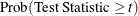and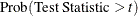for a right-sided test. The exact mid p-value is smaller and less conservative than the nonadjusted exact p-value. For more information, see Agresti (2013, section 1.1.4) and Hirji (2006, sections 2.5 and 2.11.1). For two-sample data, PROC NPAR1WAY provides mid p-values for the one-sided tests of the linear rank statistics. For multisample data, PROC NPAR1WAY provides mid p-values for the one-way ANOVA tests.

##### Computational Resources

PROC NPAR1WAY uses relatively fast and efficient algorithms for exact computations. These algorithms, together with improvements in computer power, now make it feasible to perform exact computations for data sets where previously only asymptotic methods could be applied. Nevertheless, there are still large problems that might require a prohibitive amount of time and memory for exact computations, depending on the speed and memory available on your computer. For large problems, consider whether exact methods are really needed or whether asymptotic methods might give results quite close to the exact results while requiring much less computer time and memory. When asymptotic methods might not be sufficient for such large problems, consider using Monte Carlo estimation of exact p-values, as described in the section Monte Carlo Estimation.

A formula does not exist that can predict in advance how much time and memory are needed to compute an exact p-value for a certain problem. The time and memory required depend on several factors, including which test is being performed, the total sample size, the number of rows and columns, and the specific arrangement of the observations into table cells. Generally, larger problems (in terms of total sample size, number of rows, and number of columns) tend to require more time and memory. Additionally, for a fixed total sample size, time and memory requirements tend to increase as the number of rows and columns increase, since this corresponds to an increase in the number of tables in the reference set. Also for a fixed sample size, time and memory requirements increase as the marginal row and column totals become more homogeneous. For more information, see Agresti, Mehta, and Patel (1990) and Gail and Mantel (1977).

At any time while PROC NPAR1WAY is computing exact p-values, you can terminate the computations by pressing the system interrupt key sequence (see the SAS Companion for your system) and choosing to stop computations. After you terminate exact computations, PROC NPAR1WAY completes all other remaining tasks. The procedure produces the requested output and reports missing values for any exact p-values not computed by the time of termination.

You can also use the MAXTIME= option in the EXACT statement to limit the amount of time PROC NPAR1WAY uses for exact computations. You specify a MAXTIME= value that is the maximum amount of time (in seconds) that PROC NPAR1WAY can use to compute an exact p-value. If PROC NPAR1WAY does not finish computing the exact p-value within that time, it terminates the computation and completes all other remaining tasks.

##### Monte Carlo Estimation

If you specify the MC option in the EXACT statement, PROC NPAR1WAY computes Monte Carlo estimates of the exact p-values instead of directly computing the exact p-values. Monte Carlo estimation can be useful for large problems that require a great amount of time and memory for exact computations but for which asymptotic approximations might not be sufficient. To describe the precision of each Monte Carlo estimate, PROC NPAR1WAY provides the asymptotic standard error and% confidence limits. The confidence levelis determined by the ALPHA= option in the EXACT statement; by default, ALPHA=0.01, which produces 99% confidence limits. The N= option in the EXACT statement specifies the number of samples PROC NPAR1WAY uses for Monte Carlo estimation; the default is 10,000 samples. You can specify a larger value for n to improve the precision of the Monte Carlo estimates. Because larger values of n generate more samples, the computation time increases. Or you can specify a smaller value of n to reduce the computation time.

To compute a Monte Carlo estimate of an exact p-value, PROC NPAR1WAY generates a random sample of tables with the same total sample size, row totals, and column totals as the observed table. PROC NPAR1WAY uses the algorithm of Agresti, Wackerly, and Boyett (1979), which generates tables in proportion to their hypergeometric probabilities conditional on the marginal frequencies. For each sample table, PROC NPAR1WAY computes the value of the test statistic and compares it to the value for the observed table. When estimating a right-sided p-value, PROC NPAR1WAY counts all sample tables for which the test statistic is greater than or equal to the observed test statistic. Then the p-value estimate equals the number of these tables divided by the total number of tables sampled, which can be written as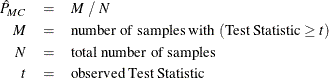PROC NPAR1WAY computes left-sided and two-sided p-value estimates in a similar manner. For left-sided p-values, PROC NPAR1WAY evaluates whether the test statistic for each sampled table is less than or equal to the observed test statistic. For two-sided p-values, PROC NPAR1WAY examines the sample test statistics according to the expression for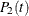given in the section Definition of p-Values.

The variable M is a binomial variable with N trials and success probability p. It follows that the asymptotic standard error of the Monte Carlo estimate is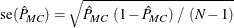PROC NPAR1WAY constructs asymptotic confidence limits for the p-values according to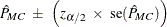where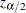is the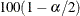percentile of the standard normal distribution, and the confidence levelis determined by the ALPHA= option in the EXACT statement.

When the Monte Carlo estimate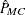equals 0, PROC NPAR1WAY computes confidence limits for the p-value as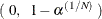When the Monte Carlo estimateequals 1, PROC NPAR1WAY computes the confidence limits as